# How does 74hc595 shift register work | Step by step instructions

### How does the 74hc595 shift register work?Hello, welcome back. In this tutorial, I will show you how does 74hc595 shift register work with Arduino. This tutorial is especially important if you want to get a lot of outputs using three PINs on an Arduino or other microcontroller. Also, this is explained using Arduino in this tutorial. For that, 8 LEDs were used. Without VCC and GND, this LED 8 is controlled by three digital pins. One shift register is used for that. You can connect these as many as you want. However, only 3 pins are required for this. You can use the knowledge in this tutorial to create an LED matrix, LED cube, etc.

### What is the 74HC595 Shift register IC and how does it work?

That is simply called 8-bit shift register IC. That is, it contains 8 bits of memory. These eight bits remain as “0” before a pulse is given.We mainly need the following 3 pins to work with this shift register ic. These are included in the shift register IC itself.
1.RCLK – Register clock
2.SRCLK – Serial clock
3.SER – Serial
The first step is to HIGH these three pins. Then, by LOW the register clock a serial communication will take place and the register will be ready. Next, the serial clock should be LOW. Then the values of the eight indicators move forward one step. It’s a memory shift. That is the value in index zero moves to the first index. Also, the value in the last index will be deleted. Otherwise, if another shift register is connected, Switch to it. This process causes the first indicator to be empty.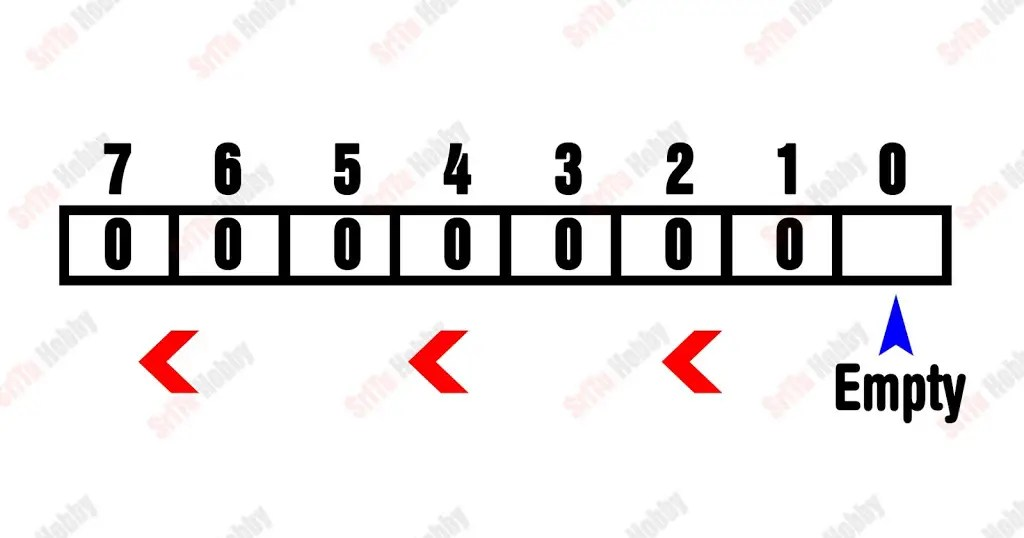You can set a value for this empty index. It should be 0 (LOW) or 1 (HIGH). For this, the serial pin can be HIGH or LOW. That is, if we HIGH the serial pin, the value of that index will be written as 1.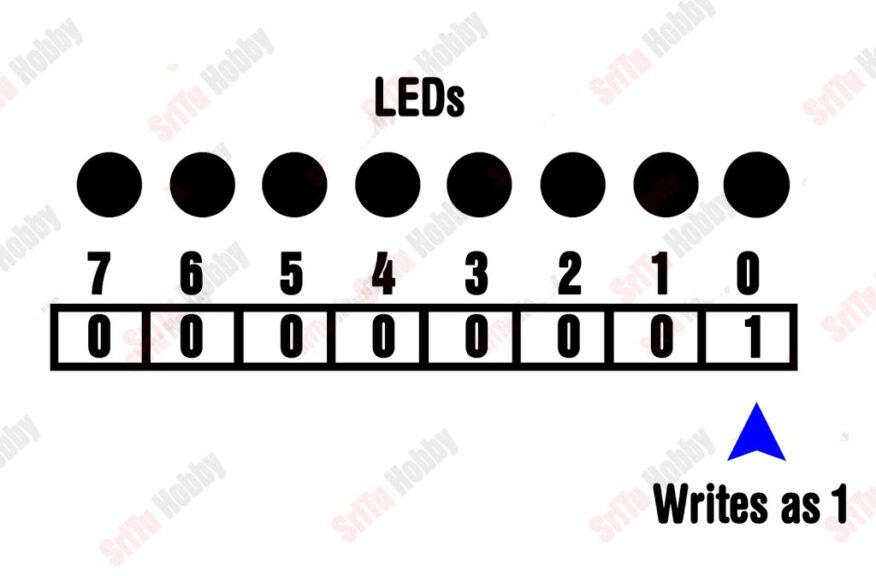Now we need to HIGH the register clock pin again to get an output from the pin corresponding to this index. That is, to light the LED bulb connected to that pin. Then the first LED bulb lights up. Continuing this process will cause the LEDs to light up one by one.

### PIN structure of this 74HC595 shift register.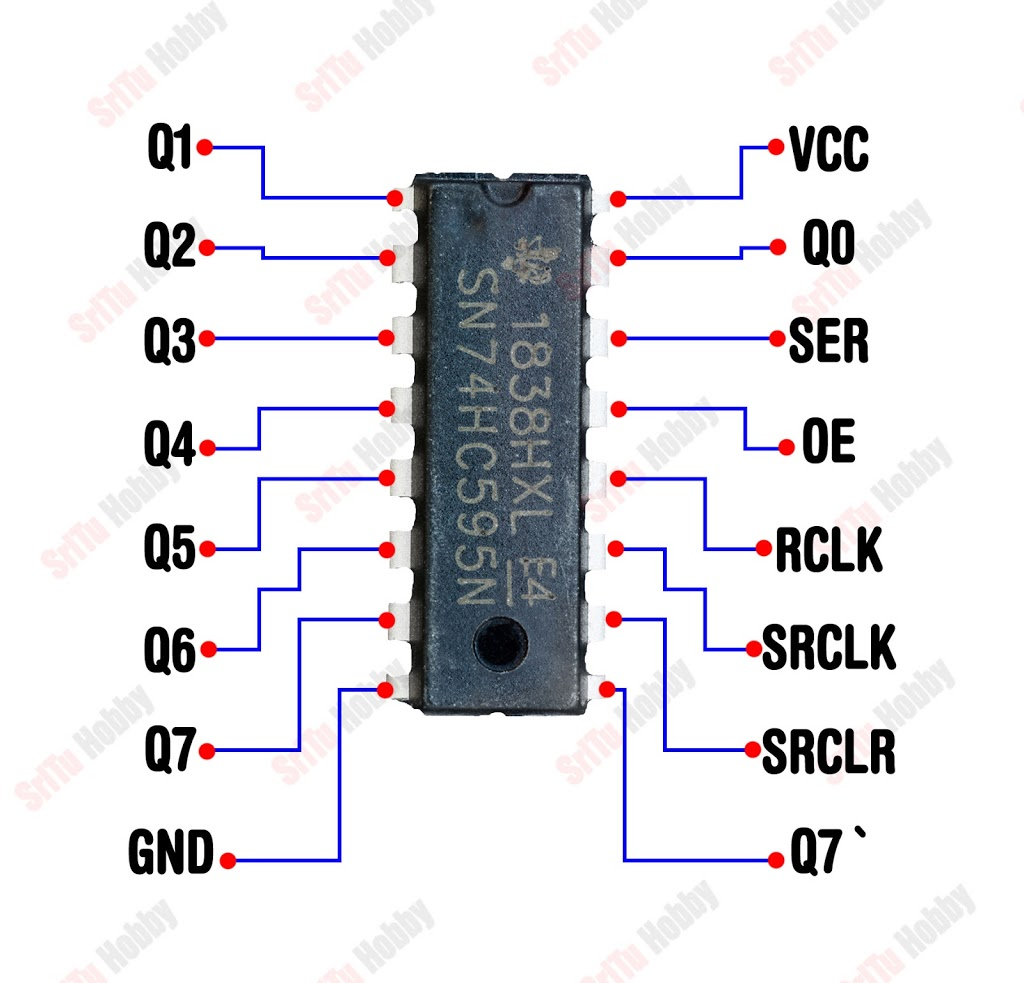• Q0 to Q7 — Output PINs.
• GND — connect to the Arduino GND.
• VCC — Connect to the VIN or 5v.
• SER — Data sending pin.
• OE — When this pin is HIGH, the output pins are disabled and current flow is prevented. Also, when this pin is low, the output pins work normally.
• RCLK — Register clock PIN (Described above)
• SRCLK — Serial clock (Described above)
• SRCLR — This pin is used to reset the memory in the shift register.
• Q7` — This pin is used to connect another shift register.
So, let’s learn how to connect this 74HC595 shift register step by step with Arduino. To do this, the required components are given below.

### Step 1

Firstly, identify these components.

#### Arduino UNO board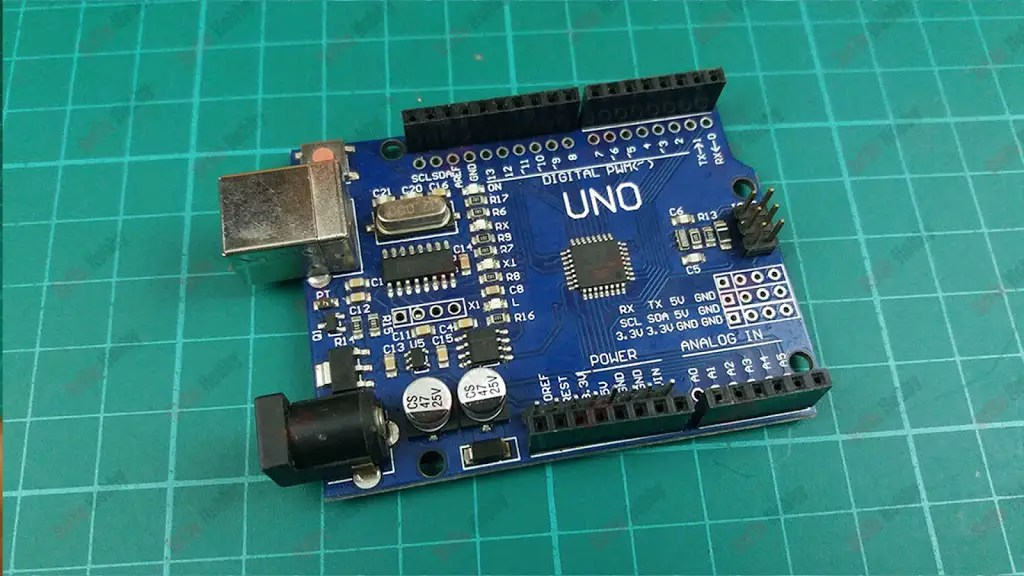#### 74HC595 shift register#### Jumper wires### Step 2

Secondly, connect these components. For that, use the circuit diagram below.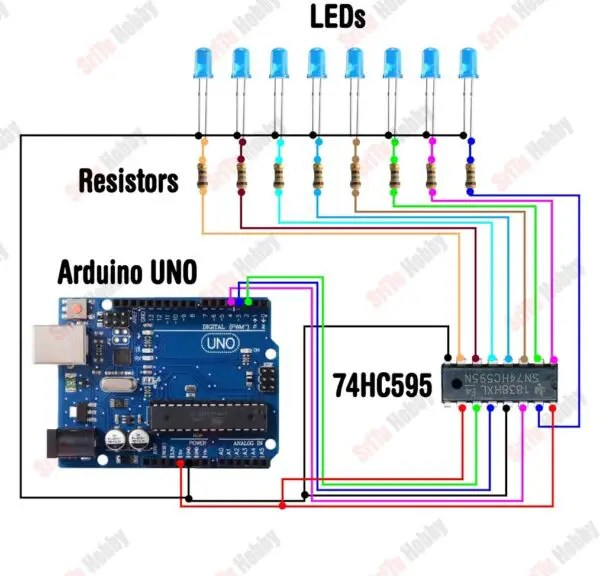### Step 3

Thirdly, let’s creates the program for this tutorial. It is as follows.
``````/*How to use 74hc595 shiftRegister.
Four LED patterns.
created by the SriTu Tech team.
Read the code below and use it for any of your creations.
*/

#include <ShiftRegister74HC595.h>//include library
ShiftRegister74HC595<1> led(4, 2, 3);//<number of shiftregisters>object name(data pin,clock pin,latch pin)

void setup() {
}

void loop() {
pattern1(2);
pattern2(2);
pattern3(2);
pattern4(2);
}

void pattern1(int a) {
for (int x = 0; x < a; x++) {
led.setAllHigh();
delay(300);
led.setAllLow();
delay(300);
}
}
void pattern2(int a) {
for (int x = 0; x < a; x++) {
for (int a = 0; a < 8; a++) {
led.set(a, HIGH);
delay(100);
led.set(a, LOW);
}
for (int b = 7; b > 0; b--) {
led.set(b, HIGH);
delay(100);
led.set(b, LOW);
}
}
}
void pattern3(int a) {
for (int x = 0; x < a; x++) {
for (int a = 0; a < 8; a++) {
led.set(a, HIGH);
delay(100);
}
for (int b = 7; b > 0; b--) {
led.set(b, LOW);
delay(100);
}
}
}
void pattern4(int a) {
for (int x = 0; x < a; x++) {
for (int a = 0; a < 8; a++) {
led.set(a, LOW);
delay(100);
led.set(a, HIGH);
}
for (int b = 7; b > 0; b--) {
led.set(b, LOW);
delay(100);
led.set(b, HIGH);
}
}
}``````

Code explanation

First, shift register library included.
#include <ShiftRegister74HC595.h>
Then, the object was created for the library. It includes shift registers quantity and data pins. For that, use the below structure.
<number of shiftregisters>object name(SER,SRCLK,RCLK)

ShiftRegister74HC595<1> led(4, 2, 3);

In the setup function, nothing is included.
void setup() {
}
In the loop function, four LED patterns are called. IF you want run these LED patterns on by one.Comment on patterns other than the required pattern.
void loop() {
pattern1(2);
pattern2(2);
pattern3(2);
pattern4(2);

}
This patterns codes are as follows.
void pattern1(int a) {
for (int x = 0; x < a; x++) {
led.setAllHigh();
delay(300);
led.setAllLow();
delay(300);
}
}
void pattern2(int a) {
for (int x = 0; x < a; x++) {
for (int a = 0; a < 8; a++) {
led.set(a, HIGH);
delay(100);
led.set(a, LOW);
}
for (int b = 7; b > 0; b–) {
led.set(b, HIGH);
delay(100);
led.set(b, LOW);
}
}
}
void pattern3(int a) {
for (int x = 0; x < a; x++) {
for (int a = 0; a < 8; a++) {
led.set(a, HIGH);
delay(100);
}
for (int b = 7; b > 0; b–) {
led.set(b, LOW);
delay(100);
}
}
}
void pattern4(int a) {
for (int x = 0; x < a; x++) {
for (int a = 0; a < 8; a++) {
led.set(a, LOW);
delay(100);
led.set(a, HIGH);
}
for (int b = 7; b > 0; b–) {
led.set(b, LOW);
delay(100);
led.set(b, HIGH);
}
}
}

### Step 4

OK, now select board and port, Afterward, upload this code to the Arduino board.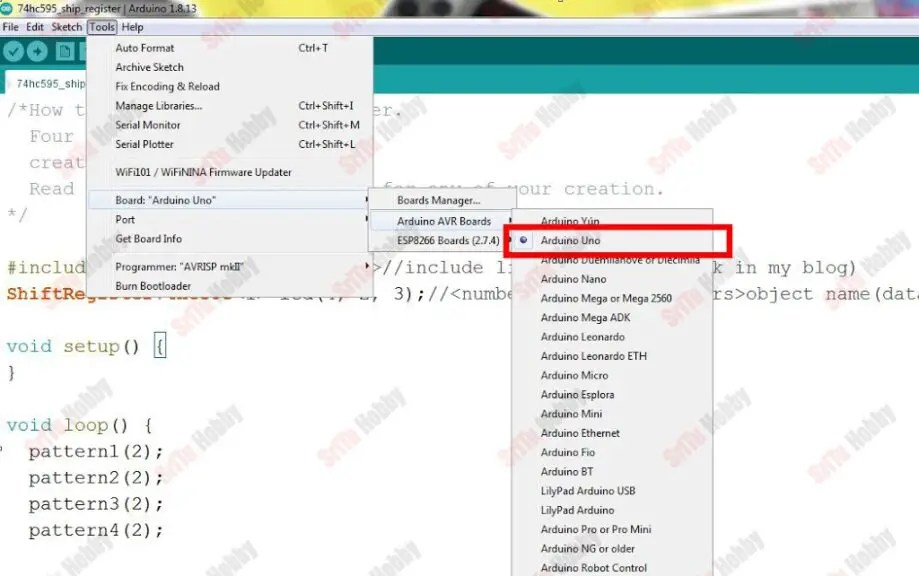OK, enjoy this tutorial. The full video guide is given below. So, we will meet in the next tutorial.
Shopping Cart
Scroll to Top